Courses

# Principle of Virtual Work for a system of connected Rigid body Mechanical Engineering Notes | EduRev

## Mechanical Engineering : Principle of Virtual Work for a system of connected Rigid body Mechanical Engineering Notes | EduRev

The document Principle of Virtual Work for a system of connected Rigid body Mechanical Engineering Notes | EduRev is a part of the Mechanical Engineering Course Engineering Mechanics.
All you need of Mechanical Engineering at this link: Mechanical Engineering

n degree of freedom systems

Having discussed single and two degree of freedom systems, and introduced the concept of generalised forces we can now consider the general case of an n degree of freedom system.

A virtual displacement must be consistent with the constraints on the system. The motion can be described by n independent, generalised co-ordinates, q1 q2 qn , ,...., . Hence a virtual displacement can be represented by small changes in these co-ordinates:-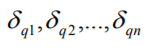Suppose only one co-ordinate, qi ( 1 ≤i ≤n) i 1 is given a small, imaginary displacement, . δ qi As a result every particle in the system will be, in general, displaced a certain amount. The virtual work done will be of the form δW = Qiδqi where Qi is an expression relating directly to the forces acting on the system. Qi is the generalised force associated with qi .

From the principle of virtual work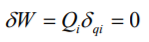Since, δqi is finite, we get

Qi=0

This must be true for i = 1,2,...,n.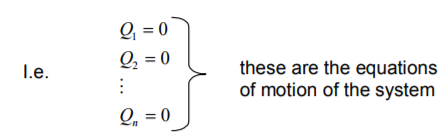The generalised forces have component parts

1) inertial forces (mass x acceleration)

2) elastic or restraining forces

3) damping forces (energy dissipation)

4) external forces

5) constraint forces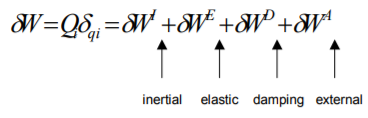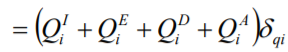(Noting, as before, that the constraint forces do no virtual work)

Then the equations of motion are:

These are the n equations of motion

We will examine each of these components now, in more detail. The aim is to relate these component forces to the generalised co-ordinates q1,q2...qn.

Inertial Forces (See also Handout)

The position of the ith particle of mass, in the system, is, in general, related to the n generalised co-ordinates, and time (if the constraints are independent of time) then the position of the ith particle depends only on the n generalised coordinates. Thus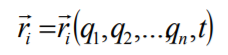(1)

Now we suppose that the system is in motion and that we represent the inertial force on the ith particle (using D’Alembert’s Principle) as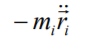(2)

We now give the system an arbitrary virtual displacement – this can be

represented in terms of generalised co-ordinates by δq1 δq2 δqn , ,..., . The virtual displacement of the Ithparticle can be represented by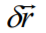(3)

and the virtual work done by the inertia force on the Ith particle is simply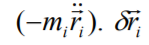(4)

(note that this is a scalar product). From this result we get the total virtual work as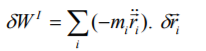(5)

Using equation (1) we have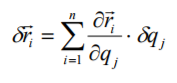(6)

Hence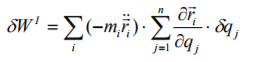(7)

and re-arranging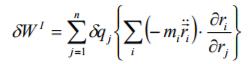(8)

However, the generalised inertial forces, Qj, are effectively defined by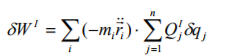(9)

Comparing (8) and (9) we have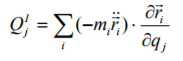(10)

It is shown in the handout notes that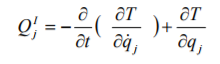(11)

where T is the total KE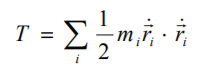(12)

Elastic Forces

Consider a simple spring: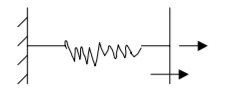For static equilibrium

FE = FS

(external force) = (internal spring force)

Suppose we define the POTENTIAL ENERGY, V, as the work done by the external force to extend the spring a distance χ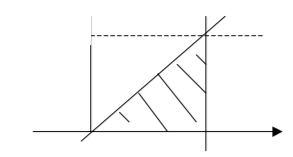external work done =1/2kx2 = V

∴ V=f(x)=V(x)− a function of x

Here V=1/2kx2

The work done by the internal spring force, W, is equal and opposite to V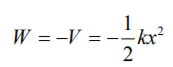Now consider a small, virtual displacement, δx . Corresponding changes to W and V are as follows: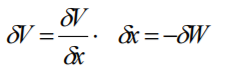Comparing with standard form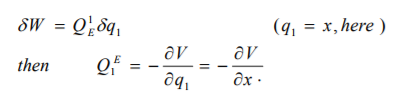Generally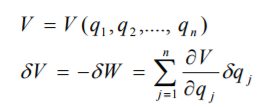Compare with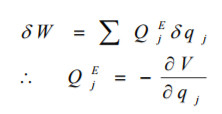Lagrange’s equation

Suppose that no damping forces are present, and there are no externally applied forces. Then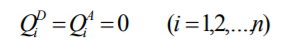We have found that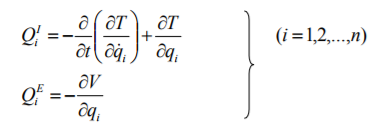If we collect these results we get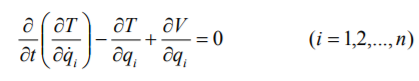This is LAGRANGE’s EQUATION

If we define

L=T-V

And assume that V does not depend on the qi & ’s, then Lagrange’s equation can be written as: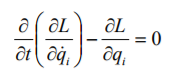Example 1- mass/spring system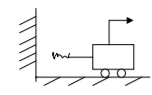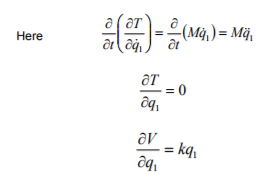This is a single degree of freedom system. Here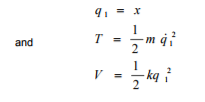The Lagrange equation is (n=1 so only one equation)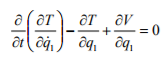Example 2-simple pendulum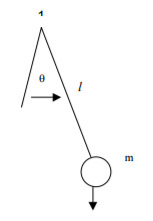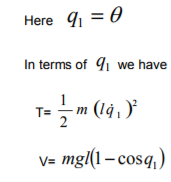Hence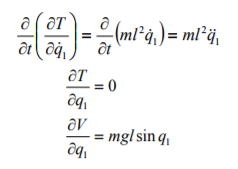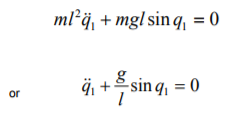Offer running on EduRev: Apply code STAYHOME200 to get INR 200 off on our premium plan EduRev Infinity!

## Engineering Mechanics

37 videos|85 docs|64 tests

,

,

,

,

,

,

,

,

,

,

,

,

,

,

,

,

,

,

,

,

,

;